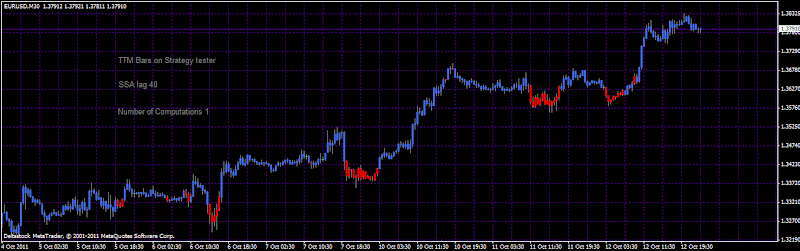# beathespread

## TTM bars with SSA end - pointed engineTTM Bars with SSA end-pointed engine

This is TTM bars with  SSA end - pointed engine. Unfortunately, this is not a reliable holy grail, from time to time repaints occur that is why I recommend to use higher level of lag bars ans low level of computations. Even if SSA end - pointed does not have a future leak this is due to some defect in the code of the TTM.

That is why the screen shot here is not when I apply but I have used the Strategy tester where I plot bar per bar. When the current bar is calculated and is plotted the already plotted bars are not changes. You may need more lag SSA bars in order to achieve a smoother result. The same if you apply less computations SSA computations you would achieve smoother results.

More lag SSA bars: Smoother results

Less lag SSA bars: Sharper results

More number of compuations: Sharper result

Less number of computations: Smoother results

Installation: If you have the  SSA end - pointed libraries do not update them they are the same. I put the libraries for ease of installation.

Trading strategy.

I did not define yet, you can replace them in any strategy with coloured bars of your choice. Maybe we should consider a strategy when there will be an entry at the change of bar colour at the close. In that way we can use an optimizer to find out what are the best parameters, and we will find if we have a robust solution. Anyway it looks nice on the chart.

SSA what is it?

Most if you have followed the big journey of the use of SSA. Check the Wikipedia:

SSA is a nonparametric spectral estimation method based on embedding a time series X(t): t = 1,N in a vector space of dimension M. SSA proceeds by diagonalizing the  lag-covariance matrix of X(t) to obtain spectral information on the time series, assumed to be stationary in the weak sense.

Basically this indicator is an idea how we can use this scientific tool as a technical analysis instrument. You do not need to understand in depth what SSA is, you need to understand what it does. It decomposie the time series and then reconstruct them.

Singular Spectrum Analysis (SSA) is a method that takes all the variability in a series and breaks it into a few oscillation patterns that we refer to as eigenvectors. It also gives a measure of significance of these patterns or eigenvalues.

“Eigen” is a German word, which roughly translates to “characteristic”. Eigenvectors are structure functions that best represent the modes of behavior in price. The eigenvalues are a measure of the variance that these modes account for. The eigenvectors can be ordered by eigenvalue, highest to lowest, so that the first few patterns retain most of the variance in the data. The ordered eigenvalues are referred to collectively as the Singular Spectrum.

You can check also the 101 of SSA here.